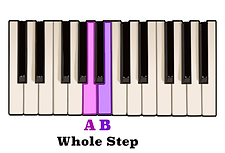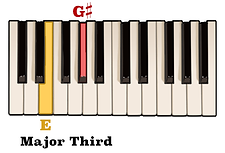top of page

## Interval Identification | Intervals Of A Third (3 of 10)

Similar to what we learned in the previous video about intervals of a second, an interval of a third can also be either Major or minor depending upon the amount of steps separating each note.

## Minor Third Intervals

For example, an interval of a minor third occurs when two notes are separated by the distance of three consecutive half steps.

Now, we know that a whole step is essentially the same as two consecutive half steps. Therefore, if two half steps equals one whole step, then three consecutive half steps may also be looked at as being comparatively the same as the combination of one whole step and one half step.

Let's use this pattern of steps to figure out what an interval of a minor third above the note A is.A whole step above the note A is the note B,and a half step above the note B is the note C.As you can see, an interval of a minor third above the note A is the note C.

By following this pattern of steps, you will be able to figure out what an interval of a minor third above any note is.

## Major Third Intervals

As mentioned in the opening video of this series, an interval of a Major third occurs when two notes are separated by the distance of four consecutive half steps. Knowing that four half steps is comparatively the same as two whole steps, let's figure out what an interval of a Major third above the note G is.Starting on the note G, one whole step takes us to the note A.Another whole step above the note A is the note B.Here we see that an interval of a Major third above the note G is the note B.

This pattern of steps which produces an interval of a Major third may also be applied to any note.

## Relation To The Scale | Minor Third Intervals

In certain situations, using the scale to figure out different intervals above a given note may be a viable option as well.

For example, we just learned that an interval of a minor third above the note A is the note C. That being said, if we take a look at an A minor scale, we can see that the third note in the scale is also the note C.In other words, an interval of a minor third above any given note is also the third scale degree of a corresponding minor scale.

With this in mind, let's figure out what an interval of a minor third above the note G is.

Looking at the notes of a G minor scale, we can see the the third scale degree, or the third note in the scale, is the note Bb. Therefore, an interval of a minor third above the note G is the note Bb.Let's double check by counting the amount of steps which produces an interval of a minor third like we learned earlier in this video.A whole step above the note G is the note A,

and a half step above A is the note Bb.

As you can see a minor third above the note G is the note Bb.

## Relation To The Scale | Major Third Intervals

This same method of using the scale may be applied to intervals of a Major third as well.

We already know that an interval of a Major third above the note G is the note B. Looking at the notes of a G Major scale we can see that the third scale degree, or the third note in the scale, is the note B.In that same respect, the third scale degree of an E Major scale is the note G#.If we follow the pattern of steps which produces an interval of a Major third, we will see that G# is in fact an interval of a Major third above the note E.

An interval of a third applies to any two notes which span three positions on the staff.

Because there are more than one step separating each note, when reading intervals of a third on the staff you will notice that each note will always go from either a line directly to the next line above or below it,or from a space directly to the next space above or below it.Knowing this makes identifying intervals of a third on the staff relatively simple. However, just like intervals of a second, it is up to the performer to determine whether the distance separating each note is an interval of a minor third, or an interval of a Major third.

bottom of page